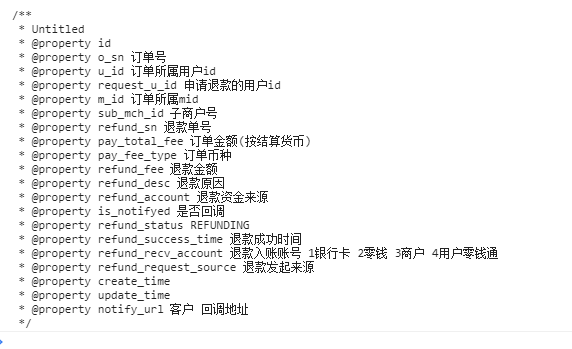# 类与对象 - PHP手册笔记

+关注继续查看

## 基本概念

PHP对待对象的方式与引用和句柄相同，即每个变量都持有对象的引用，而不是整个对象的拷贝。

<?php
$instance = new stdClass();$assigned = $instance;$reference = & $instance;$instance->var = '$assigned will have this value.';$instance = null;
var_dump($instance); var_dump($reference);
var_dump($assigned); 这段代码的输出如下，这是为什么呢？ null null object(stdClass) public 'var' => string '$assigned will have this value.' (length=31)

PHP 5.3引进了两个新方法来创建一个对象的实例，可以使用下面的方法创建实例。

<?php
class Test {
static public function getNew() {
return new static;
}
}
class Child extends Test {}
$obj1 = new Test();$obj2 = new $obj1; var_dump($obj1 !== $obj2); // true$obj3 = Test::getNew();
var_dump($obj3 instanceof Test); // true$obj4 = Child::getNew();
var_dump($obj4 instanceof Child); // true var_dump($obj1 == $obj2); // true PHP不支持多重继承，被继承的方法和属性可以通过同样的名字重新声明被覆盖，注意参数必须保持一致，当然构造函数除外。但是如果父类定义方法时使用了final，则该方法不可被覆盖。可以通过parent::来访问被覆盖的方法和属性，parent::只能访问父类中的常量const，不能访问变量。 <?php class A { private$name = 'A';
const conname = 'A';
public function getName() {
return $this->name; } } class B extends A { private$name = 'B';
const conname = 'B';
public function getName() {
return $this->name; } public function getParent() { return parent::conname; } } class C extends B { private$name = 'C';
const conname = 'C';
public function getName() {
return $this->name; } public function getParent() { return parent::conname; } }$a = new A;
var_dump($a->getName()); // A$b = new B;
var_dump($b->getName()); // B var_dump($b->getParent());  // A
$c = new C; var_dump($c->getName());  // C

## 自动加载类

<?php
function __autoload($class_name) { require_once$class_name.'.php';
}
$obj1 = new MyClass1();$obj2 = new MyClass2();

## 构造函数和析构函数

PHP 5允许开发者在一个类中定义一个方法作为构造函数，构造函数也不支持重载。

## 访问控制

<?php
Class Test {
private $foo; public function __construct($foo) {
$this->foo =$foo;
}
private function bar() {
echo 'Accessed the private method.';
}
public function baz(Test $other) {$other->foo = 'hello';
var_dump($other->foo);$other->bar();
}
}
$test = new Test('test');$test->baz(new Test('other'));

## 抽象类

PHP 5支持抽象类和抽象方法。类中如果有一个抽象方法，那这个类必须被声明为抽象的。

<?php
abstract class AbstractClass {
abstract protected function prefixName($name); } class ConcreteClass extends AbstractClass { public function prefixName($name, $separator = ', ') { if($name === "Pacman") {
$prefix = 'Mr'; } elseif($name === 'Pacwoman') {
$prefix = "Mrs"; } else {$prefix = '';
}
return "$prefix$separator $name "; } }$class = new ConcreteClass;
echo $class->prefixName('Pacman'); echo$class->prefixName('Pacwoman');

## traits

<?php
trait A {
public function smallTalk() {
echo 'a';
}
public function bigTalk() {
echo 'A';
}
}
trait B {
public function smallTalk() {
echo 'b';
}
public function bigTalk() {
echo 'B';
}
}
class Talker {
use A, B {
B::bigTalk as talk;
}
}
$t = new Talker();$t->smallTalk();  // b
$t->bigTalk(); // A$t->talk();  // B

<?php
trait HelloWorld {
public function sayHello() {
echo 'Hello World.';
}
}
class MyClass1 {
use HelloWorld {
sayHello as protected;
}
}
class MyClass2 {
use HelloWorld {
sayHello as private myPrivateHello;
}
}

<?php
trait Hello {
public function sayHelloWorld() {
echo 'Hello ' . $this->getWorld(); } abstract public function getWorld(); } class MyHelloWorld { private$world;
use Hello;
public function getWorld() {
return $this->world; } public function setWorld($val) {
$this->world =$val;
}
}
$c = new MyHelloWorld;$c->setWorld('world');
$c->sayHelloWorld(); 如果trait定义了一个属性，那类将不能定义同样名称的属性，否则会产生错误。 ## 重载 PHP提供的重载是指动态地创建类属性和方法，与其它绝大多数面向对象语言不同。通过魔术方法来实现。当使用不可访问的属性或方法时，重载方法会被调用。所有的重载方法都必须被声明为public 使用__get()__set()__isset()__unset()进行属性重载，示例如下。 <?php class PropertyTest { private$data = array();
public $declared = 1; private$hidden = 2;
public function __set($name,$value) {
echo "Setting $name to$value. " . '<br>';
$this->data[$name] = $value; } public function __get($name) {
echo "Getting $name. <br>"; if(array_key_exists($name, $this->data)) { return$this->data[$name]; } return null; } public function __isset($name) {
echo "Is $name set? <br>"; return isset($this->data[$name]); } public function __unset($name) {
echo "Unsetting $name. <br>"; unset($this->data[$name]); } }$obj = new PropertyTest;
$obj->a = 1; var_dump($obj->a);
var_dump(isset($obj->a)); unset($obj->a);
var_dump(isset($obj->a)); var_dump($obj->declared);
var_dump($obj->hidden); 输出结果如下： Setting a to 1. Getting a. int 1 Is a set? boolean true Unsetting a. Is a set? boolean false int 1 Getting hidden. null 在对象中调用一个不可访问方法时，__call()会被调用。用静态方式中调用一个不可访问方法时，__callStatic()会被调用。参数为调用方法的名称和一个枚举数组，注意区分大小写。 使用__call()__callStatic()对方法重载，示例如下。 <?php class MethodTest { public function __call($name, $arguments) { echo "Calling object method$name " .
implode(', ', $arguments) . '<br>'; } public static function __callStatic($name, $arguments) { echo "Calling static method$name " .
implode(', ', $arguments) . '<br>'; } }$obj = new MethodTest;
$obj->runTest('in object context'); MethodTest::runTest('in static context'); ## 遍历对象 对象可以用过单元列表来遍历，例如用foreach语句。默认所有可见属性都将被用于遍历。 <?php class MyClass { public$var1 = 'value 1';
public $var2 = 'value 2'; public$var3 = 'value 3';
private $var4 = 'value 4'; protected$var5 = 'value 5';
}
$obj = new MyClass; foreach($obj as $key =>$value) {
echo "$key =>$value <br>";
}

## 魔术方法

PHP 将所有以__（两个下划线）开头的类方法保留为魔术方法。定义类方法时，除魔术方法外，建议不要以__为前缀。

__sleep__wakeup不清楚具体做什么用的，示例程序中给出了个数据库连接的例子。

__toString方法用于一个类被当成字符串时应怎样回应。此方法必须返回一个字符串，且不能再方法中抛出异常。如果将一个未定义__toString()方法的对象转换为字符串，将产生错误。

## 对象比较

<?php
class Base {}
class A extends Base {}
class B extends Base {}
$a = new A;$b = new B;
var_dump($a ==$b);  // false

## 后期静态绑定

<?php
class A {
public static function who() {
echo __CLASS__;
}
public static function test() {
self::who();
}
}
class B extends A {
public static function who() {
echo __CLASS__;
}
}
B::test();  // A
B::who();  // B

static::关键字表示运行时最初调用的类，后期静态绑定就是这样使用。如下面程序所示，也就是说调用test()时引用的类是B而不是A

<?php
class A {
public static function who() {
echo __CLASS__;
}
public static function test() {
static::who();
}
}
class B extends A {
public static function who() {
echo __CLASS__;
}
}
B::test();  // B
B::who();  // B

<?php
class A {
public static function foo() {
static::who();
}
public static function who() {
echo __CLASS__;
}
}
class B extends A {
public static function test() {
A::foo();
parent::foo();
self::foo();
}
public static function who() {
echo __CLASS__;
}
}
class C extends B {
public static function who() {
echo __CLASS__;
}
}
C::test();  // ACC

## 对象序列化

（全文完）PHP 简单 对象 (object) 与 数组 (array) 的转换
PHP 简单 对象 (object) 与 数组 (array) 的转换
0 0php对象字段声明,easyswoole ORM 快速生成注释

0 0PHP中对象的序列化和反序列化
serialize() 返回字符串，可以存储于任何地方。 serialize() 可处理除了 resource 之外的任何类型。甚至可以 serialize() 那些包含了指向其自身引用的数组。 这有利于存储或传递 PHP 的值，同时不丢失其类型和结构。 在需要恢复的地方使用unserialize()函数即可
0 0php echo对象,php __toString
php echo对象,php __toString
0 0PHP：empty判断对象为空
PHP：empty判断对象为空
0 0PHP：json_encode转换成json空列表和空对象
PHP：json_encode转换成json空列表和空对象
0 0Python/PHP/JS对象与json数据的转换key顺序问题
Python/PHP/JS对象与json数据的转换key顺序问题
0 0PHP方法类与对象的区别
PHP方法类与对象的区别
0 0

PHP进阶教程 - 由浅入深掌握面向对象开发 - 第一阶段20332

PHP进阶教程 - 由浅入深掌握面向对象开发 - 第二阶段13633

PHP进阶教程 - 由浅入深掌握面向对象开发 - 第三阶段20831

PHP完全自学手册文档教程940588

Java面向对象开发23594106

C语言学习教程18823128

PHP安全开发_从白帽角度做安全

PHP在机器学习上的应用及云深度学习平台的架构设计与实现

PHP与APM_技术内幕和最佳实践